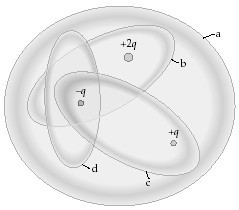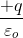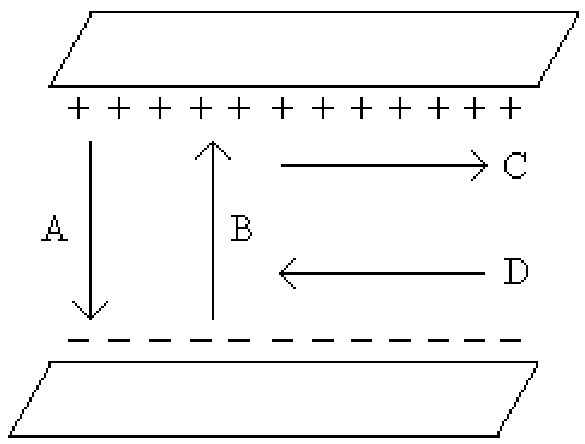College Physics : Gauss's Law

Example Questions

Example Question #1 : Gauss's Law

A conductor is placed in an electric field under electrostatic conditions. Which of the following statements is correct for this situation?

The electric field on the surface of the conductor is perpendicular to the surface

The electric field is zero inside the conductor

All valence electrons go to the surface of the conductor

All of these

All of these

Explanation:

A conductor is defined as a object free to move charges. In particular, valence electrons, which are the outer most electron in each atom and the most free to move, travel inside the conductor until the net electric field inside the conductor is zero. These electrons will move until this condition has been met. Because of the presents of charged particles at the surface and the condition that they are no longer moving, any electric field at the surface must be perpendicular to that surface.

Example Question #1 : Gauss's LawThe figure shows four Gaussian surfaces surrounding a distribution of charges. Which Gaussian surfaces have an electric flux ofthrough them?

A

B

B and D

C

B

Explanation:

Gauss' laws states that the the electric flux through a Gaussian surface will be proportional to the net total charge inside the Gaussian surface. By inspection of the figure, we see that Gaussian surface B is the correct answer.

Example Question #1 : Gauss's LawThe Figure shows four Gaussian surfaces surrounding a distribution of charges. Which Gaussian surfaces have no electric flux through them

B

A

C

B and D

C

Explanation:

According to Gaussian law, the electric flux will be zero when the net electric charge inside the Gaussian surface is zero. By inspection, we see this is Gaussian surface C.

Example Question #3 : Gauss's Law

Gaussian surfaces A and B enclose the same positive charge +Q. The area of Gaussian surface A is three times larger than that of Gaussian surface B. The flux of electric field through Gaussian surface A is __________.

equal to the flux of electric field through Gaussian surface B

unrelated to the flux of electric field through Gaussian surface B

three times smaller than the flux of electric field through Gaussian surface B

nine times larger than the flux of electric field through Gaussian surface B

equal to the flux of electric field through Gaussian surface B

Explanation:

According to Gauss's law, the total electric flux is equal to the net total electric charge inside the a Gaussian surface. If the Gaussian surface is three times larger, the electric flux will be the same if both Gaussian surfaces contain the same amount of total electric charge.

Example Question #1 : Gauss's LawWhich of the arrows shown in the figure represents the correct direction of the electric field between the two metal plates?

D

C

A

B